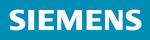### Short Circuit Calculations Spreadsheets No.1

Siemens - Estimated Available Fault Current Calculation Spreadsheet1- Input data:

the input Data for this Fault Current Calculations spreadsheet will be:

• Select the secondary voltage of used transformer.
• Sum the total horsepower of all motors on system (optional but recommended).
• Provide AIC (Ampere Interrupting Capacity) if the transformer is inside your system, or if the utility available current is known.
• Enter the primary voltage to the transformer if available (used only of you have provided a value for the available primary fault current).
• Enter the transformer rating in KVA.

• Input the nameplate transformer impedance as a percentage, not a decimal (i.e. 5.5% would be 5.5 not to be 0.055).
• OR If you have the available fault current, either given to you from utility, or if you are using this program to calculate fault currents at other parts of the system where the AIC (Ampere Interrupting Capacity) is known, input this number and it will automatically override the transformer based information.
• Input the conductor length in feet (one way length).
• Select the conductor’s size.
• Select the conductor material (Aluminum or copper).
• Select the method of conductor’s installation (in conduit or in cable).
• Select the type of the used raceway/cable (steel or non-magnetic).

2- Output data:the Output data from this Fault Current Calculations spreadsheet will be:
• The rated secondary current in Amperes
• Available fault current

Note: This spreadsheet estimates available fault currents for three phase systems. The calculation of motor contribution in this calculator is approximate only – systems with large motor contribution, high X/R conditions, and closed transition paralleled sources or high impedance grounding will require a more accurate calculation method.